Get instant live expert help with Excel or Google Sheets“My Excelchat expert helped me in less than 20 minutes, saving me what would have been 5 hours of work!”

#### Post your problem and you'll get expert help in seconds

Your message must be at least 40 characters
Our professional experts are available now. Your privacy is guaranteed.

# Nth Root of a Number in Excel

There are two ways to find the nth root of any number in Excel. These are using the POWER function or using the caret ^ ”. This tutorial will walk through each method.

## Find the nth root of a number in Excel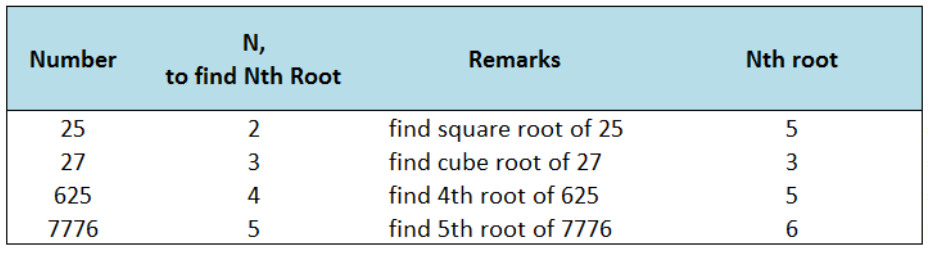Figure 1. Sample table showing the nth root of a number

### Setting up the Data

Create below table with Number, N and Nth Root as headers, and a remarks column for clarification.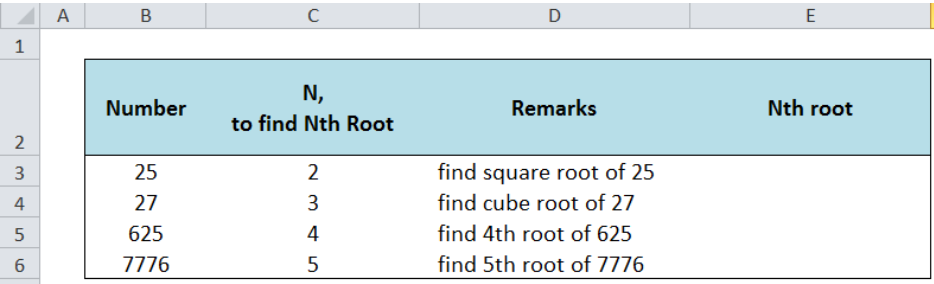Figure 2. Sample data for finding nth root of a number

### Nth Root using the POWER Function

The POWER function returns the result of a given number that is raised to a specified power.

### Syntax

`=POWER(number, power)`

Where

• Number: the base number we want to raise to a certain power
• Power is the exponent to which the base number is raised;
• When finding the nth root of a number, the power is presented as “ 1/n ”

In cell E3, enter the formula:

`=POWER(B3,1/C3)`

This formula calculates the square root of 25, which is 5.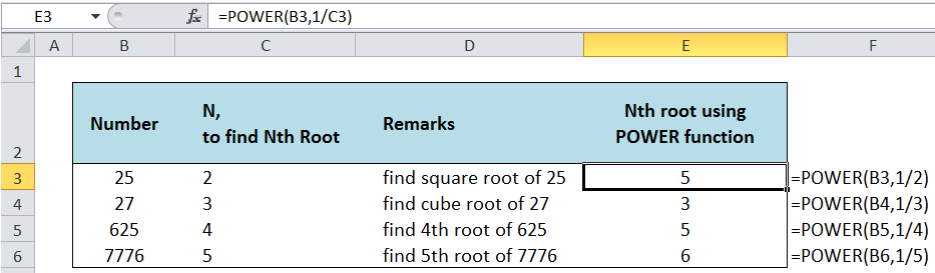Figure 3. Using POWER function to find nth root of a number

Through the POWER function, we can find the nth root of any number in Excel.

### Nth Root using Caret “^” in Excel

The caret symbol “^” in Excel means “to the power of”.  It provides a quicker way to compute for the nth root of any number.

### Syntax

`=number^(power)`

In cell E3, enter the formula:

`=B3^(1/C3)`

Similar to the POWER function, this formula calculates the square root of 25, which is 5.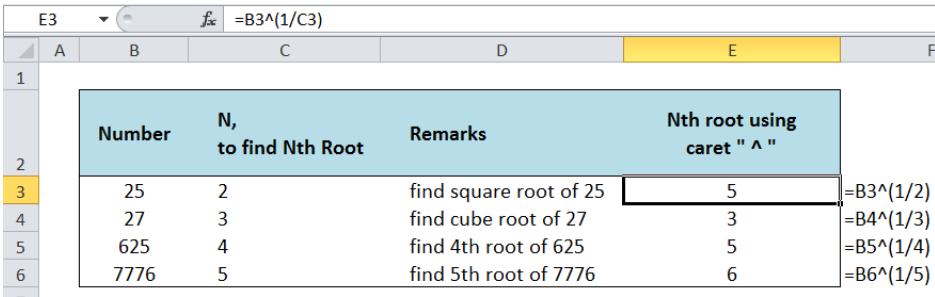Figure 4. Using caret “^” to find the nth root of a number

Below table compares the use of POWER function and the caret “^” in finding the nth root of a number.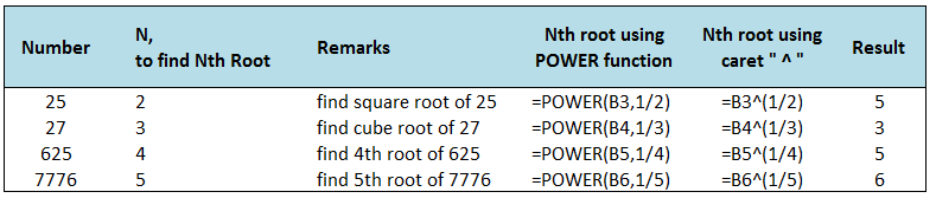Figure 5. POWER function and caret “^” returning the same results

Most of the time, the problem you will need to solve will be more complex than a simple application of a formula or function. If you want to save hours of research and frustration, try our live Excelchat service! Our Excel Experts are available 24/7 to answer any Excel question you may have. We guarantee a connection within 30 seconds and a customized solution within 20 minutes.

### Did this post not answer your question? Get a solution from connecting with the expert.Another blog reader asked this question today on Excelchat:
Related blogs## Subscribe to Excelchat.coAnother blog reader asked this question today on Excelchat: